## Overview

This guide introduces Arm Helium technology, which is the M-profile Vector Extension (MVE) for the Arm Cortex-M processor series. The Arm Cortex-M55 processor is the first Arm processor to support this technology.

Helium is an extension of the Armv8.1-M architecture and delivers a significant performance uplift for Machine Learning (ML) and Digital Signal Processing (DSP) applications for small, embedded devices.

Helium technology provides optimized performance by using Single Instruction Multiple Data (SIMD) to perform the same operation simultaneously on multiple data items. This can be particularly effective in DSP and ML.

At the end of this guide:

• You will be familiar with Helium and the differences it has with Neon
• Helium registers
• Predication in Helium
##### Before you begin

You should have familiarity with the Arm architecture, see our Introducing the Arm architecture for more information.

## What is Helium?

Arm Helium technology is the M-Profile Vector Extension (MVE) for the Arm Cortex-M processor series. Helium is an extension of the Armv8.1-M architecture and delivers a significant performance increase for Machine Learning (ML) and Digital Signal Processing (DSP) applications.

Helium lets you optimize performance by using Single Instruction Multiple Data (SIMD) to perform the same operation simultaneously on multiple data items. The Helium instructions are designed for Digital Signal Processing (DSP) and Machine Learning (ML) applications in particular.

##### Data processing methodologies

A common technique to accelerate workloads that process many data elements at the same time with one instruction is to exploit opportunities for parallelism that are often available. This data processing methodology is called Single-Instruction Multiple-Data (SIMD). Processing elements sequentially and one at a time is called Single-Instruction Single-Data (SISD). In this section of the guide, we will discuss both operating on multiple data elements at the same time reduces the total time that is required to process all data elements. A reduced compute time means that results are available at a higher throughput and lower latency, and that the CPU can go in a low power state early to save energy. With Armv8.1-M, the SIMD data processing methodology is supported with the Arm Helium technology.

##### Single Instruction Single Data

Most Arm instructions are Single Instruction Single Data (SISD). Each instruction performs its specified operation on a single data item. Processing multiple data items therefore requires multiple instructions.

The following example shows how to perform four addition operations. You can see that this requires four instructions to add values from four pairs of registers:

```ADD r0, r0, r5
```

This method is slow, and it can be difficult to see how different registers are related.

If the values that you are dealing with, are smaller than the maximum register size, the extra potential bandwidth is wasted with SISD instructions. For example, when adding 8-bit values together, each 8-bit value must be loaded into a separate 32-bit register. Performing many individual operations on small data items does not use machine resources efficiently.

##### Single Instruction Multiple Data

Single Instruction Multiple Data (SIMD) instructions perform the same operation for multiple data items. These data items are packed as separate lanes in a larger register.

For example, the following instruction adds four pairs of 32-bit values together. However, in this case, the values are packed into separate lanes in two pairs of 128-bit registers: the q registers. Each lane in the first source register is then added to the corresponding lane in the second source register, before being stored in the same lane in the destination register. You can see this in the following code:

```VADD.I32 q2, q1, q0
// This operation adds two 32-bit (word) lanes, q0 and q1,
// and stores the result in q2.
// Each of the four 32-bit lanes in each register is added separately.
// There are no carries between the lanes.
```

This single instruction operates on all data values in the large register, as you can see here: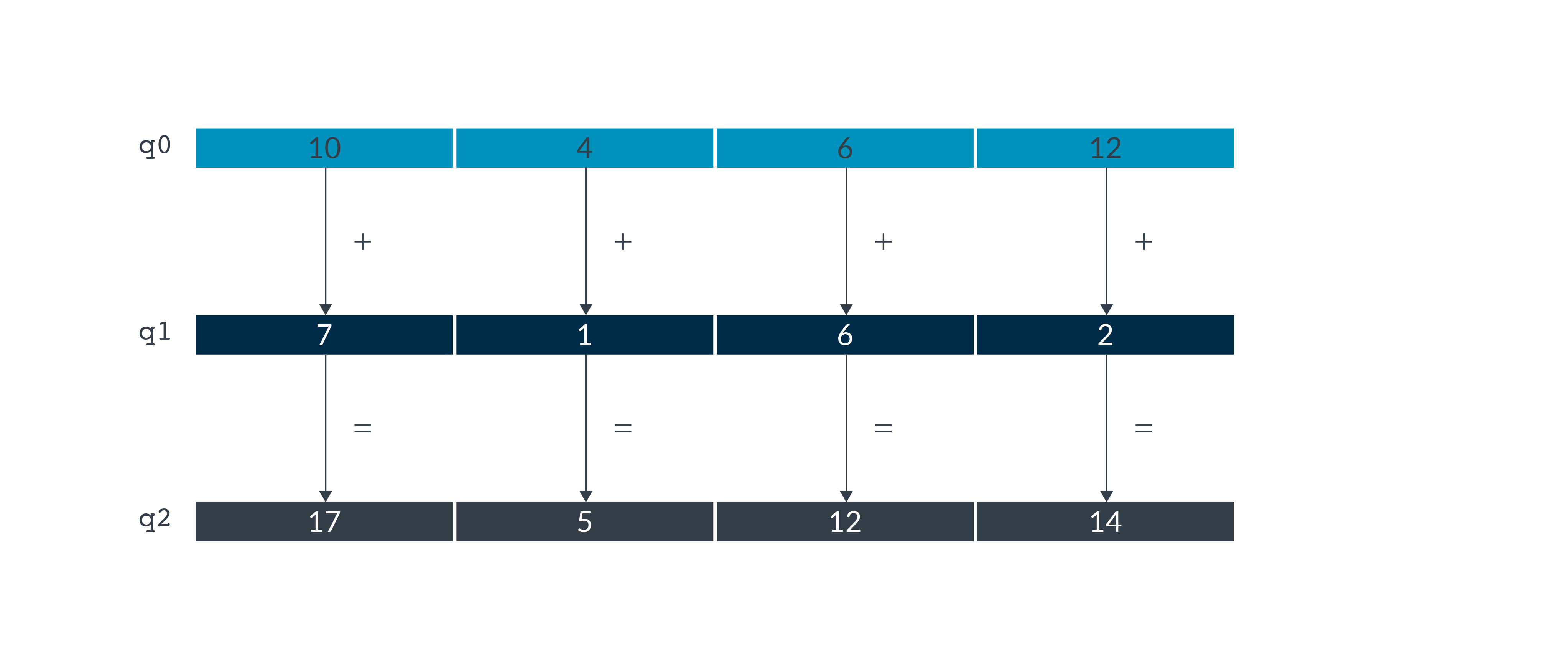Being able to specify parallel operations in a single instruction like this allows the processor to do the calculations simultaneously, which increases performance and throughput. The preceding diagram shows 128-bit registers, with each register holding four 32-bit values. Operations on data elements with different data sizes are possible for Helium registers. We explain this in more detail in Helium registers

The addition operations that are shown in the diagram are independent for each lane. For example, any overflow or carry from lane 0 does not affect lane 1. Lane 1 is an entirely separate calculation.

Media processors, like audio and video devices, often split each full data register into multiple registers and perform computations on the registers in parallel. If the processing for the data sets is simple and repeated many times, SIMD can give considerable performance improvements. It is beneficial for digital signal processing, and multimedia algorithms, for example:

• Audio, video, and image-processing codecs
• 2D graphics based on rectangular blocks of pixels
• 3D graphics
• Color-space conversion
• Physics simulations
• Machine Learning
• Convolutions
• Clustering
##### Helium and Neon comparison

One of their main differences between Helium and Neon is that Helium is the extension that is used for the Armv8.1-M architecture. Neon is the extension that is used for the Armv7-A architecture.

The similarities between Helium and Neon are:

• Both use 128-bit vectors.
• Both reuse floating point registers.
• Both provide instructions to perform vector add and vector multiply.

The differences between Helium and Neon are:

• Helium includes eight vector registers in Helium and Neon includes 16 vector registers.
• Helium includes both regular registers and vector registers. This allows Helium to reduce the register pressure.
• Helium supports some new data types, for example, fp16, which is available in Armv8.2-A. Neon does not support these data types.
• Helium includes features like loop predication and lane predication that Neon does not include.
##### Applications

Two of the main applications for Helium are Digital Signal Processing (DSP) and Machine Learning (ML). Helium offers significant performance increase in these areas.

Helium provides intrinsics targeted for DSP instructions, for example:

• `vld2q`, which loads blocks of data from memory and writes them to the destination registers
• `vrmlsldavhaq` is useful for the multiplication of complex numbers, which is often used in DSP.

ML is a subset of Artificial Intelligence (AI) that provides systems the ability to automatically learn and improve from experience without being explicitly programmed. Helium helps to boost Matrix Multiplication operations, which are the foundation of Convolutional Neural Networks or Classical based Machine Learning kernels.

Applications that can be greatly accelerated by Helium are Fast Fourier Transform (FFT) and Complex Dot Product as there are specific instructions which help implement these calculations.

## Helium registers

##### Registers, vectors, lanes, and elements

The Helium registers contain vectors of elements of the same data type. The same element position in the input and output registers is referred to as a lane.

Usually each Helium instruction results in noperations, where nis the number of lanes that the input vectors are divided into. Each operation is contained within the lane.

The number of lanes in a Helium vector depends on the size of the vector and the data elements in the vector.

A 128-bit Helium vector can contain the following element sizes:

• Two 64-bit integers
• Four 32-bit integers or single precision float
• Eight 16-bit integers or half precision float
• Sixteen 8-bit integers

Elements in a vector are ordered from the least significant bit to the most significant bit. That is, element 0 uses the least significant bits of the register. Let’s look at an example of a Helium instruction. The instruction `VADD.16 q0, q0, q5` performs a parallel addition of eight lanes of 16-bit (8 x 16 = 128) integer elements from vectors in `q5` and `q0`, storing the result in `q0`. This can be seen in the following diagram: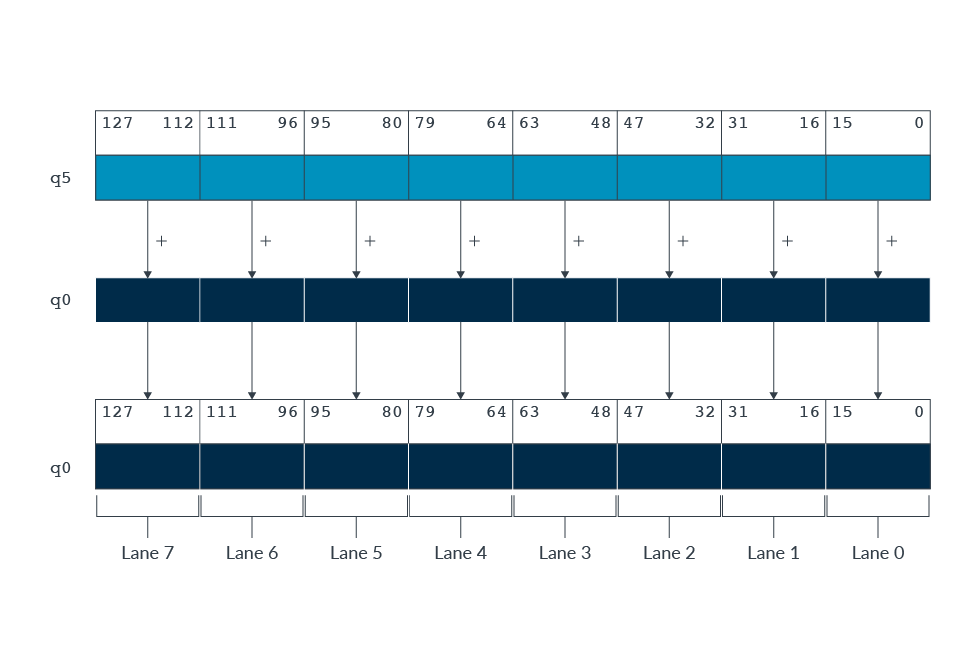Helium instructions use a mix of vector and scalar operands, including:

• Vector by vector to vector
• Vector by scalar to vector
• Vector by vector to scalar

For example, multiplication, this can be seen in Vector instruction example. You can find more examples in the Armv8-M Architecture Reference Manual

##### Data types

When programming for Helium in C or C++, different data types let you declare vectors of different sizes. To use these data types, we must add the library `arm_mve.h` to the program. This header file provides data types that look like the following:

• Sixteen 8-bit elements = `int8x16, uint8x16, float8x16`
• Eight 16-bit elements = `int16x8, uint16x8, float16x8`
• Four 32-bit elements = `int32x4, uint32x4, float32x4`
• Two 64-bit elements = `int64x2, uint64x2, float64x2`
##### Predication register

Predication lets you selectively perform mathematic operations on lanes in a vector. The predication mask specifies which lanes are processed, by setting bits to true (1) or false (0). The predication status and control register, `VPR.P0`, contains this predication mask. We explain this in Helium instructions.

## Helium instructions

This section provides a brief overview of some of the instructions in Helium.

Helium registers discussed the different lane configurations that can be used in Helium registers. Helium instructions cannot execute correctly unless they are aware of the configuration of the data within the vector register. This data is encoded within the instruction itself. Helium instructions provides 20 scalar instructions and 130 vector instructions.

##### Helium instruction naming rules

The names of Helium instructions follow a common pattern. This pattern lets you determine information about the instruction operation from its name.

The following syntax shows the common pattern:

Note: Some of the letters are overloaded, but their position makes the meaning unambiguous.

`V[<mod>]<op>[<shape>][<extra>][<cond>][<.dt>] [<dest>, <src>...]`
`V`
Short for vector. Present on all the assembly instructions
`mod`
Short for modifier, for example `None`, `Q` (sat), `H` (halving), and `D` (doubling)
`op`
Short for operation, for example, `ADD`, `MUL`, `MIN`
`shape`
Indicates an extension, for example, None, L (long), or N (narrow)
`extra`
Indicates an instruction-specific modifier, for example, `None`, `T` (top), `B` (bottom), `V` (across)
`cond`
Short for conditional which is used for predication in Helium
`.dt`
Short for data type, for example, Float, Integer, Signed, Unsigned
`dest`
Indicates the destination of the vector registers, which is where the results get put
`src`
Indicates the source vector register(s), which is where the input comes from

The common pattern can be seen with examples for the MOV instruction which you can see here:

• `VMOVN<T><v>.<dt> Qd, Qm`
• In this example, the shape is N, which performs a narrowing to half-width of each data element before it is written to the destination register.
• `VQMOVN<T><v>.<dt> Qd, Qm`
• In this example, the mod is Q, which Performs an element-wise saturation to half-width.
##### Helium instruction set

Helium includes a variety of instructions and each of these instructions perform different operations, for example math operations like add, multiply, and subtract. Some of these instructions are particularly suited for DSP or ML, for example the intrinsic `vmladavaq`. `vmladavaq` is where there are two vectors and the corresponding lanes in each of these vectors are multiplied together. The result from the multiplication is added all together and the scalar value is added to this value. These math operations may all use matrix operations. Matrix operations are helpful for DSP. This is because these operations allow DSP chips to digitize sounds or images that are stored or transmitted electronically.

##### Different types of load and store

Helium provides three different types of load and store instructions:

This is the most straightforward way to load data into vectors. With continuous load, each lane or memory is accessed in sequence, starting from a base address that is specified in a scalar register. For example, `VLDRB` (Vector Load Register Byte), loads consecutive bytes from memory into a destination vector register.
Widening or narrowing
If data that is stored in memory is a different size to the vector lanes, then widening or narrowing is required. This is usually required for packing or unpacking input or output data. An example is the instruction `VLDRB.U32` (Vector Load Register Byte). This instruction loads consecutive bytes from memory and will zero-extend the byte to 32 bits and then place it into the corresponding lane in the destination vector.
Scatter or Gather
Scatter or Gather involves gathering data from non-contiguous locations. For example, when manipulating one color channel of RGB data, you gather data items from every third memory location and load them into a vector register.
Generators are the `viddup`, `vddup`, `viwddup`, and `vdwdup` instructions. These instructions generate incrementing or decrementing index sequences intended to be passed to gather or scatter load and stores. For example:
```// 16-bit vector gather load with decrementing offsets with a step of 2
mov            r0, #20                // decrementing sequence start
vddup.u16      q0, r0, #2             // Generator, decrement step of 2
// q0 = [  20 18  16 14  12 10  8 6  ]
vldrh.s16      q1, [r1, q0, uxtw #1]  // gather load, base = r1, offset = q0
```
##### Different types of math operations
 Math Operation Description Basic arithmetic Integers are used in arithmetic such as addition and subtraction. This is used in many of the predication examples that will be explained in Predication. Complex arithmetic Instructions like add or multiply (`VCMLA`) operate on real and imaginary components in the same vector. This might be used in, for example, Fast Fourier transforms (FFTs). MAC operators Operands are multiplied and accumulated. We explained one of these instructions, `VMLA`, in Vector instruction example. This might be used in, for example, matrix multiplication.

## Predication

Predication provides a way to conditionally execute a block of instructions, on lanes that meet specified criteria. The predication mask specifies which lanes are processed by setting bits to true (1) or false (0).

Each bit represents the predication of a lane in the 128-bit Helium vector. Therefore, when using vectors which contain a lane width of 32 bits, 4 out of the 16 bits control predication. The following table shows different lane widths:

 Lane width Bits in `VPR.P0` 32 bits [12, 8, 4, 0] 16 bits [14, 12, 10, 8, 6, 4, 2, 0] 8 bits [15:0]

There are four different types of predication intrinsics:

`_m`
Merging
`_z`
Zeroing
`_x`
Don't care
`_p`
Predicated

The different types of predication can be used for loop tail handling. When input data is not a multiple of 128-bits, the final loop iteration needs to process a partially empty vector. For example, a data array of ten 32-bit values is processed as two full iterations on vectors containing four elements, and a final loop iteration on a vector containing two elements. Merging predication loads a value from the inactive vector into these lanes. Zeroing predication can mark the unused lanes on the final loop iteration. Pruning predication can only store the values in the lanes that are set to true. Don’t care predication can be used when we don’t care whether an undeclared or declared value are loaded into the unused lanes.

##### Examples of predication
Merging
Merging predication can be used for clipping values in a vector that exceed a specified maximum. Merging predication is where false predicated lanes, are filled with the corresponding element from the inactive vector.
The intrinsic `vaddq_m` is an example of a merging predication. `vaddq_m` adds two vectors together, and the false predicated lanes are filled with the value from the inactive vector. This is explained in the following example:
`int32x4_t [__arm_]vaddq_m[_s32] (int32x4_t inactive, int32x4_t a, int32x4_t b, mve_pred16_t p)`
In this example, the four inputs are:
• `Inactive`
• A 32x4 vector which contains 4,4,4,4
• `a`
• A 32x4 vector which contains 5,2,3,6
• `b`
• A 32x4 vector which contains 7, 1, 6, 2
• `p`
• A predicate mask, containing 16 bits. For 32-bit lanes, the bits in the mask which control lane predication are bits 12, 8, 4, and 0. In this example, bits 12 and 1 have been set to true and bits 8 and 4 have been set to false, resulting in a predicate mask of 0001000000000001.
The output vector is stored in`inactive`. This example uses a vector that is 32x4. The vector could be a different size. Helium registers has more information. However, the vector registers; inactive, a and b must contain the same number of lanes.
Looking at the lane predication in detail, we can see that:
• Bits 8 and 4 control lanes two and three. In our example, these bits contain zero. This means that lanes two and three take their value from the inactive vector. In both cases this value is four.
• Bits 12 and 0 control lanes one and four. In our example these bits contain one. This means that lanes one and four take their value from the result of adding the corresponding lanes in the vectors a and b.
• For bit 12 this corresponds to 5+7 = 12
• For bit 0 this corresponds to 6+8 = 14
Therefore, the result vector equals: 12,4,4,14.
This process is illustrated in the following diagram: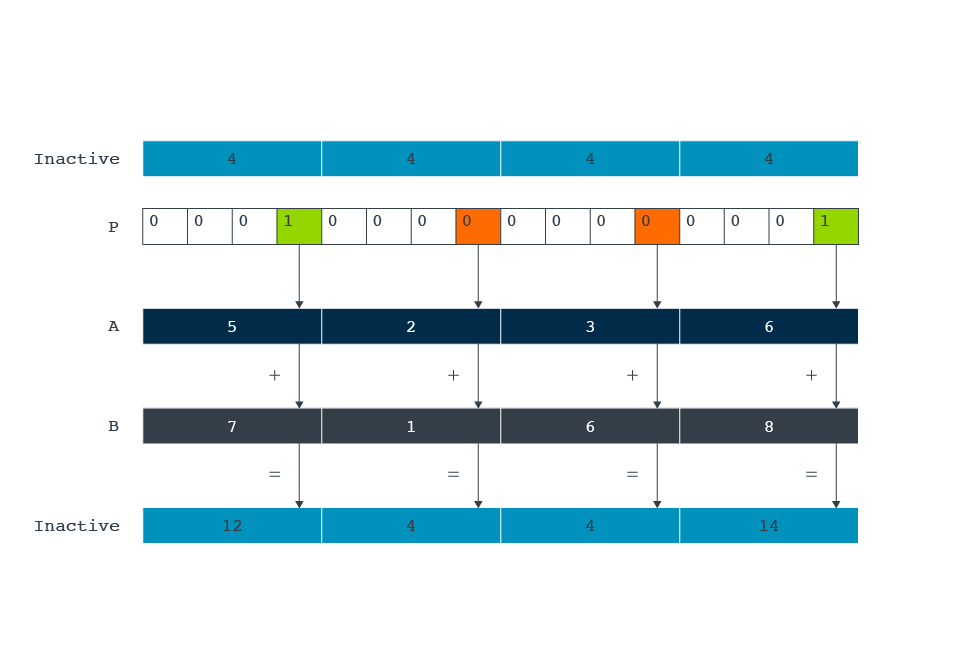The following assembly code assumes that r0 points to the `0x11111111 0x22222222 0x33333333 0x44444444` pattern in memory. The following code is an example of merging predication:
```// predicated addition (inactive lanes untouched)
mov             r1, 0xf00f      // set mask for 32-bit elements 0 & 3
vmsr            p0, r1          // set predicate bit 0 & 4
vldrw.s32       q0, [r0]        // q0 = { 0x11111111 0x22222222 0x33333333 0x44444444}
movw            r2, 0x5555
movt            r2, 0x5555
vdup.32         q1, r2          // q1 = {0x55555555 0x55555555 0x55555555 0x55555555}
vpst
vaddt.i32       q1, q0, q0      // q1 = {0x22222222 0x55555555 0x55555555 0x88888888}
```
```movw            r2, 0x0000      // set upper bound = 0x30000000
movt            r2, 0x3000
vldrw.s32       q0, [r0]        // q0 = { 0x11111111 0x22222222 0x33333333 0x44444444}
vpt.s32         ge, q0, r2      // enable lanes greater or equal than r2
vdupt.32        q0, r2          // set q0[i] to r2 for active lanes, others are untouched
// q0 = { 0x11111111 0x22222222 0x30000000 0x30000000}
```
Zeroing
Zeroing predication is used for load instructions. The false predicated lanes are set to zero.
The following intrinsic is an example of a zeroing predicated load, which loads consecutive elements from memory into a destination vector register:
`uint32x4_t [__arm_]vldrwq_z[_s32] (uint32_t const * base, mve_pred16_t p)`
Consider an example where the two inputs are:
• `Base`
• A pointer to the start of an array containing 32-bit unsigned integer values. In this example, it contains 5, 2, 3, 6.
• `p`
• A predicated mask. For 32-bit lanes, the bits in the mask which control lane predication are bits 12, 8, 4, 0. In this example, it equals 0000000000010001.
The output is a vector containing the result.,br> Looking at the lane predication in detail:
• Four numbers are contained within memory. These numbers are loaded into a vector through base, the pointer to the array.
• Bits 12 and 8 control lanes four and three. In our example, these bits contain zero. This means that lanes four and three are populated with the value zero.
• Bits 4 and 0 control lanes two and one. In our example, these bits contain one. This means that lanes one and two are populated with the value from memory.
Therefore, the result vector equals 0, 0, 2, 5.
This process is illustrated in the following diagram: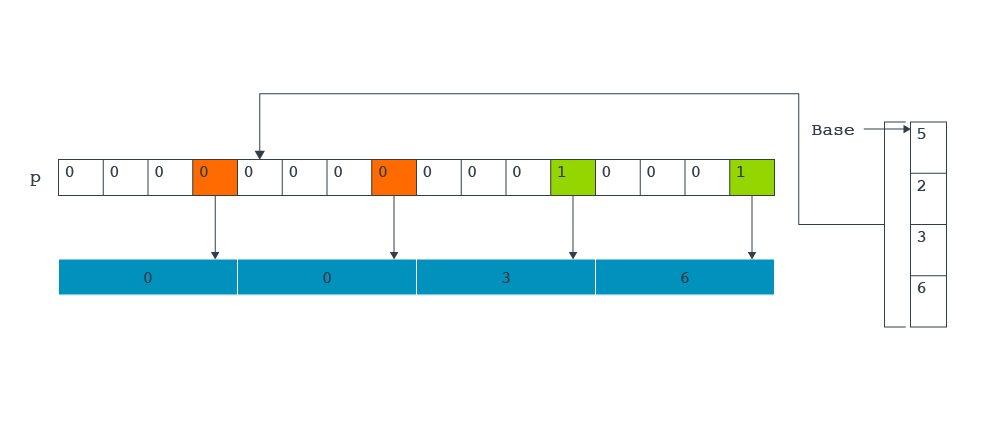The following assembly code assumes that `r0` points to the `0x11111111 0x22222222 0x33333333 0x44444444` pattern in memory. The following code is an example of zeroing predication:
```// starting with predicated load (zeroing of inactive lanes)
mov             r1, 0x0ff0      // set mask for 32-bit elements 1 & 2
vmsr            p0, r1          // set predicate bits
vpst                            // activate predication for the next slot
vldrwt.s32      q0, [r0]        // q0 = { 0x00000000 0x22222222 0x33333333
```
```// 8-bit load
mov             r1, 0x1111      // set mask for 8-bit elements 0, 4, 8, 12
vmsr            p0, r1          // set predicate bits
vpst                            // activate predication for the next slot
vldrbt.s8       q0, [r0]        // q0 = { 0x11 0x00 0x00 0x00 0x22 0x00 0x00 0x00 0x33 0x00 0x00 0x00 0x44 0x00 0x00 0x00}
```
```// 16-bit load
mov             r1, 0x300c      // set mask for 16-bit elements 1 & 6
vmsr            p0, r1          // set predicate bits
vpst                            // activate predication for the next slot
vldrht.s16      q0, [r0]        // q0 = { 0x0000 0x1111 0x000 0x00000 0x000 0x00000 0x4444 0x0000}
```
Don't care
Don’t care predication is like zeroing predication, because it is used for load instructions. The difference between don’t care predication and zeroing predication is that, when a lane has been set to false, an undeclared variable is left undefined instead of having a 0 as the output.
Predicated
Predicated predication is used when a scalar output is returned. False-predicated lanes are not used when computing the output.
The following intrinsic is an example of predicated predication. This intrinsic finds the minimum value of the elements in a vector, then compares that minimum value to the specified value a. The intrinsic returns the smaller of the two values:
`int32_t [__arm_]vminvq_p[_s32] (int32_t a, int32x4_t b, mve_pred16_t p)`
Consider an example in which the three inputs are:
• `a`
• A scalar value that equals 4
• `b`
• A 32x4 vector that contains the values: 5, 2, 3, 6
• `p`
• A predicated mask. For 32-bit lanes, the bits in the mask which control lane predication are bits 12, 8, 4, 0. In this example, it equals 0001000000000001
The output is the smaller of a or the minimum value in`b`.
Looking at the lane predication in detail:
• Bits 8 and 4 controls lanes two and three. In our example, these bits contain zero. This means that these lanes are not used.
• Bits 12 and 0 control lanes one and four. In our example, these bits contain one. This means that lanes one and four are compared to see which contains the smallest number. In this example, the smallest number is 5
• The minimum value: 5 is compared with the scalar value (`a`), which is 4.
• Therefore, the intrinsic returns 4.
This process is illustrated in the following diagram: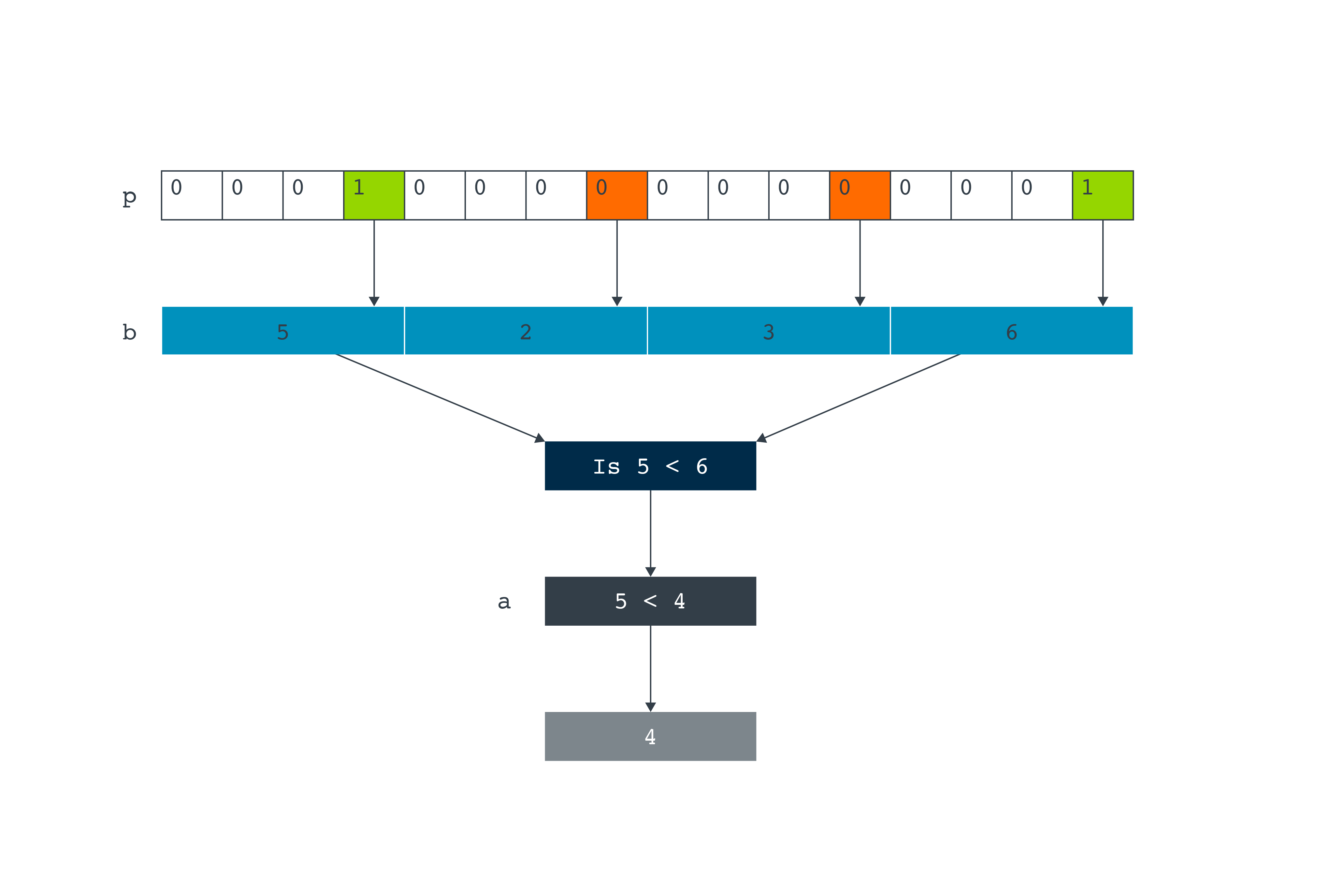The following assembly code assumes that `r0` points to the `0x11111111 0x22222222 0x33333333 0x44444444` pattern in memory. The following code is an example of predicated predication:
```// predicated 32-bit MAC (inactive lanes ignored)
mov             r1, 0x00ff      // set mask for 32-bit elements 0 & 1
vmsr            p0, r1          // set predicate bits
vldrw.s32       q0, [r0]        // q0 = { 0x11111111 0x22222222 0x33333333 0x44444444}
clrm            {r2, r3}
vpst
vrmlaldavht.s32  r2, r3, q0, q0 // r2,r3 = r2:r3 + (sum(q0[i] * q0[i]) + (1<<7)) >> 8         i={0,1}
//  (0x11111111 ^2 + 0x22222222 ^ 2  + (1<<7)) >> 8
```

## Vector instruction example

In this section of the guide, we introduce how Helium uses vector instructions when performing parallel arithmetic on vectors using vector registers. We also show how we can use intrinsics to perform parallel arithmetic.

##### Overview of Vector Multiply Accumulate

The example that we have chosen to show parallel arithmetic is Vector Multiply Accumulate (`VMLA`). At a high-level, in `VMLA` each element in the source vector is multiplied by a scalar value. The result is added to the respective element from the destination vector. The results are stored in the destination register.

Let’s look at the following `VMLA` instruction:

`VMLA.S32 VectorOne, VectorTwo, Scalarvalue`

This instruction shows that `Qda` is the accumulator vector register and is the destination register for the entire operation.

`VMLA` has three inputs and one output. The three inputs are:

• `VectorOne`
• The accumulator vector register
• `VectorTwo`
• The source vector register
• `Scalarvalue`
• The scalar general-purpose register

In the example, the vector registers, `VectorOne` and `VectorTwo`, are divided into four lanes of 32-bit values. However, the vector registers could be divided into two 64-bit values, or eight 16-bit values or sixteen 8-bit values, depending on which data types you are operating on. Both `VectorOne` and `VectorTwo` must contain the same number of data lanes.

In our example, the inputs:

• `VectorOne` contains the numbers 7,1,6,2
• `VectorTwo` contains the numbers 5,2,3,6.
• `Scalarvalue` contains 2.

When the three inputs have been declared:

• `Scalarvalue` is multiped with Qn.
• `VectorTwo` is added to Q1.
• `VectorOne` is outputted.

That is, `VectorOne[i] = VectorOne[i] + VectorTwo[i] * Scalarvalue where i={0..elts-1}`

These steps are shown in the following diagram: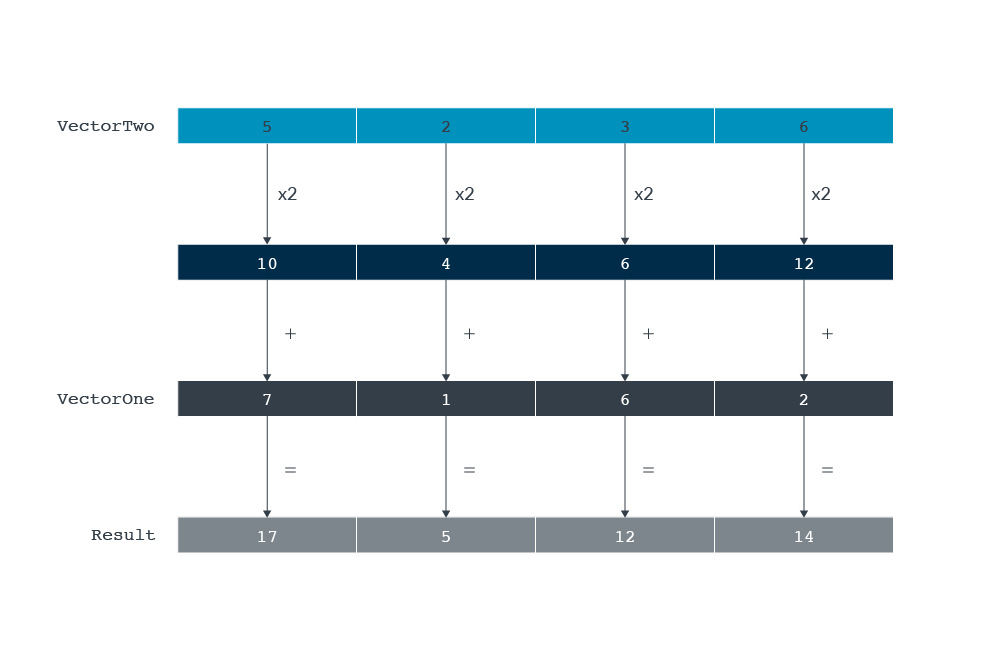##### Implementation using intrinsics

One way that you could use `VMLA` in your C code is by using intrinsics. Intrinsics are functions which the compiler understands and replaces with low-level Helium instructions.

First, we declare two arrays to hold the input vectors and an integer variable to hold the scalar value. You can see this in the following code:

```//Declaring the arrays and the scalar value
const int arrayone[] = {5, 2, 3, 6};
const int arraytwo[] = {7, 1, 6, 2};

int32_t Scalarvalue = 2;
```

In this example, we only use four numbers in our vectors. However, in a real-world example more than four numbers may be used. No matter how many numbers are in the array, pointers are needed because the intrinsic specification uses them to access the input and output values. This can be seen in the following code:

```//Declaring the pointer value
int32_t *pone = arrayone;
int32_t *ptwo = arraytwo;
```

When the arrays, and the pointers, have been declared, the values can be loaded into vector registers.

The following code uses intrinsics to load the array values into helium vector registers:

```//Loading the 4 values from the array
int32x4_t VectorOne = vld1q_s32 (pone);
int32x4_t VectorTwo = vld1q_s32 (ptwo);
```

In the following code this performs the multiply and accumulate operation:

`int32x4_t Result = vmlaq_n_s32 (VectorTwo, VectorOne, Scalarvalue); `

This is stating Result = VectorOne + (VectorTwo x Scalarvalue).

Here is a complete working example:

```#include <stdio.h>
#include <stdlib.h>
#include "arm_mve.h"

int main(void) {
printf("Program started\n");
//Declaring arrays
const int32_t arrayone[] = {5, 2, 3, 6};
const int arraytwo[] = {7, 1, 6, 2};
const int inactivearray[] = {4,4,4,4};
const int m[] = {8,8,8,8};

//Value that arraytwo is being multiplied by
int32_t scalarvalue = 2;

//pointer values for both arrays (need this because otherwise it would print out 	4 every time)
int32_t *pone = arrayone;
int *ptwo = arraytwo;

int32x4_t VectorOne = vld1q_s32 (pone);
int32x4_t VectorTwo = vld1q_s32 (ptwo);

//The VMLA instruction
int32x4_t Result = vmlaq_n_s32 (VectorTwo, VectorOne, scalarvalue);

//Printing the results
printf("Element 0: %d\n", vgetq_lane_s32 (Result,0));
printf("Element 1: %d\n", vgetq_lane_s32 (Result,1));
printf("Element 2: %d\n", vgetq_lane_s32 (Result,2));
printf("Element 3: %d\n", vgetq_lane_s32 (Result,3));
}
```
##### How this can be done using C code

In the previous sections of this guide, we introduced the multiply-accumulate instruction and how it can be generated from intrinsics. Now let’s look at the multiply-accumulate instruction can be generated from C source-code. We use the following motivating example:

```void vmla (int *__restrict Qda, int *__restrict Qn, int Rm, int N) {
for (int i = 0; i<N; i++)
Qda[i] += Qn[i] * Rm;
}
```

The preceding code shows that the C implementation is an almost direct translation of the pseudo-code that is shown in the previous section, `Qda = Qda + (Qn x Rm)`. In the preceding example, the first two arguments of the function `VMLA` are integer pointers modeling the two input streams. The first one is also the output stream. The arguments are annotated with the `__restrict` keyword to indicate that streams `Qda` and `Qn` do not overlap. For more details on `__restrict` see Arm Compiler toolchain Compiler Reference. The third argument is the scalar value `Rm`. The fourth argument is N which determines how many numbers will be processed, In the previous section and example this was 4, here it is a run-time value N.

This example also shows that writing C code has advantages compared to writing intrinsics. C code is more compact, readable, and portable. However, writing C code relies on the compiler to efficiently translate your code into machine instructions. When more fine-grained control of the generated instructions is required, intrinsics might be a better solution.

When this `VMLA` function is compiled with Arm Compiler 6.14, the following assembly code is generated:

```    dlstp.32 lr, r3
.LBB0_1:
vldrw.u32 q0, [r1], #16
vldrw.u32 q1, [r12], #16
vmla.u32 q1, q0, r2
vstrw.32 q1, [r0]
mov r0, r12
letp lr, .LBB0_1
```

Let’s look first at the generated function body that follows label `LBB0_1`. There are two load instructions loading 16 bytes in vector registers >`q0` and `q1`. Vector `q1` is multiplied by the scalar value in `r2`, which contains function argument Rm, and accumulated in vector registers `q0`. The results are then stored to `r0`, which corresponds to function argument `Qda`. The first and last instruction in this example are instructions that control the execution of the loop, which we will be discussed in Tail-predication.

The `VMLA` instruction uses vector registers, we have shown that we are generating vector code from C-code that is using scalar values and operations with `Qda[i] += Qn[i] * Rm`. For example, auto-vectorization by the compiler can transform scalar code to vector code in an efficient way. Auto-vectorization is enabled with optimization level –Os and above.

Arm Compiler User Guide: Selecting optimization options helps to transform existing code and serves as an alternative to writing (vector) intrinsics.

In Tail-predication, we discuss the last interesting aspect of the generated code example: the loop control instructions.

##### Tail-predication

In Predication, we mention tail-predication as one of the predication forms introduced in Armv8.1. The assembly code example in How this can be done using C code shows usage of two of these new instructions:

• `DLSTP`: Do-Loop-Start, Tail-Predicated
• `LETP`: Loop-End, Tail-Predicated

These two instructions are the tail-predication version of the Do-Loop Start (DLS) and Loop-End (LE) instructions and are part of the low-overhead-branches extension that aim to speed-up loop execution. Tail-predication is best illustrated with an example:

```for (int i=0; i < 10; i++)
A[i] += B[i] + C[i];
```

In this example, the loop iterates ten times, which means that it is processing 10 integer elements. If we can vectorize this code and can pack four integer elements in one vector, we have two vector operations processing eight elements. Because we need to process ten elements, we need a scalar loop (a tail loop), that processes the remaining two elements. In pseudo-code. The vectorized code looks like this:

```for (int i=0; i < 8; i+=4)       // the vector loop
A[i:4] += B[i:4] * C[i:4];
for (int i=8; i < 10; i++)       // the tail-loop
A[i] += B[i] * C[i];
```

The vector loop increments with four. It processes four 4 elements at the same time, which is indicated with the `i:4` array index notation. The tail loop is the original loop, except that it starts at 8. This means that it executes only the last 2 iterations, so `I=8, I=9`, the ninth and tenth iterations respectively.

Having both the vector and the tail loop comes at a cost, which is the overhead of executing 2 loops, and code density. Tail-predication solves these problems and allows the execution of these loops. For example, loops that process several elements. Where number of elements being processed are not an exact multiple of the number of elements that fit in a vector, in one single vector loop. In pseudo-code, that looks like this:

```    for (int i=0; i < 12; i+=4)       // the vector loop and the tail-loop
A[i:4] += B[i:4] * C[i:4],       active lane if i<10
```

The loop bound has been adjusted to 12, and the step size is 4, so that this loop executes 3 iterations. Executing 3 iterations of this vector loop would process 12 elements, while we need to process only 10. For the last iteration, tail-predication means that the last 2 lanes are disabled to make sure we only process these 10 elements and not 12. In the previous pseudo code example, this is indicated with the `active lane if i<10` annotation. The Armv8.1-M tail-predication loop instruction solves this in hardware.

Let’s now look again at the assembly output in the How this can be done using C code and the tail-predicated loop. The loop is set up with the following instruction:

`dlstp.32 lr, r3`

The instruction dlstp sets up a tail-predicated loop, where register lr contains the number of elements to be processed, with its initial value coming from register r3. This makes sense if we remember what the VMLA function prototype looks like:

`    void vmla (int *__restrict Qda, int *__restrict Qn, int Rm, int N)`

Function argument N corresponds to the number of elements that are processed by the loop, is the fourth function argument and is passed in register r3. After this, the loop body is executed, and we branch back to the beginning with new instruction, which looks like:

`letp lr, .LBB0_1`

This Loop-End (LE) instruction branches back to label. `LBB0_1` and decrements the number of elements to be processed in register `lr`. It also ensures that, for the last iteration, the right vector lanes are enabled or disabled, for example, it takes care of the tail-predication. Using Arm Compiler 6, tail-predicated loops can be generated from source code or intrinsics.